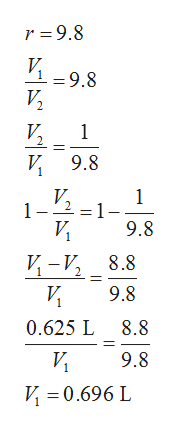# At the beginning of the compression process of an air standard Otto cycle, p1 = 1 bar, T1 = 300 K. The maximum temperature in the cycle is 2250 K and the compression ratio is 9.8. The engine has 4 cylinders and an engine displacement of Vd = 2.5 L.  Determine per cylinder:a)    the volume at state 1.b)    the air mass per cycle.c)    the heat addition per cycle, in kJ.d)    the heat rejection per cycle, in kJ.e)    the net work per cycle, in kJ.f)     the thermal efficiency.g)    the mean effective pressure, in bar.h)    Develop a full exergy accounting per cycle, in kJ. Let T0 = 300 K, p0 = 1 bar.i)    Devise and evaluate the exergetic efficiency for the cycle.

Question
750 views
At the beginning of the compression process of an air standard Otto cycle, p1 bar, T1 = 300 K. The maximum temperature in the cycle is 2250 K and the compression ratio is 9.8. The engine has 4 cylinders and an engine displacement of V= 2.5 L.  Determine per cylinder:

a)    the volume at state 1.
b)    the air mass per cycle.
c)    the heat addition per cycle, in kJ.
d)    the heat rejection per cycle, in kJ.
e)    the net work per cycle, in kJ.
f)     the thermal efficiency.
g)    the mean effective pressure, in bar.
h)    Develop a full exergy accounting per cycle, in kJ. Let T0 = 300 K, p1 bar.
i)    Devise and evaluate the exergetic efficiency for the cycle.
check_circle

Step 1

Hey, since there are multiple sub parts posted, we will answer first three sub parts. If you want any specific aub part to be answered then please submit that sub part only or specify the sub part number in your message.

Step 2

Determine the swept volume.

Step 3

Write the expression of compres...help_outlineImage Transcriptioncloser =9.8 9.8 V2 1 V9.8 1 VP 1 -2 1 -1- 9.8 И, И -и, 8.8 И 9.8 0.625 L 8.8 И 9.8 0.696 L fullscreen

### Want to see the full answer?

See Solution

#### Want to see this answer and more?

Solutions are written by subject experts who are available 24/7. Questions are typically answered within 1 hour.*

See Solution
*Response times may vary by subject and question.
Tagged in

### Mechanical Engineering